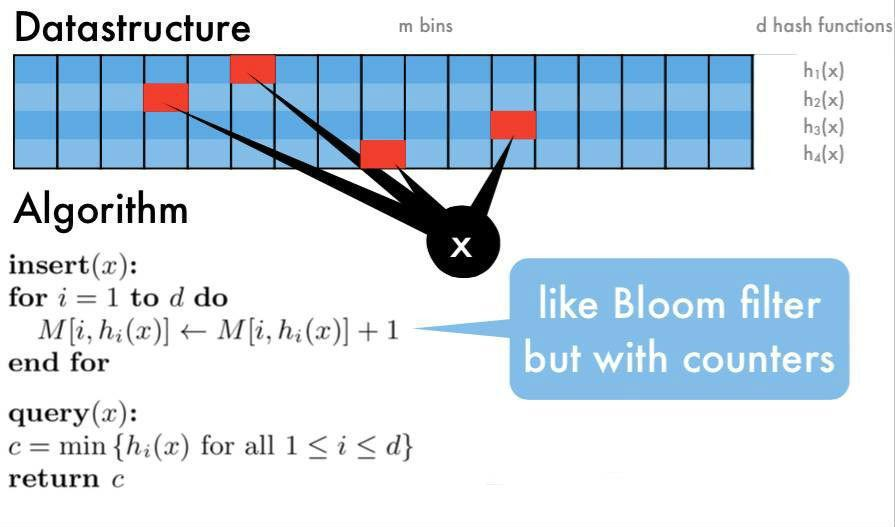Top K frequent elements is a classic interview question that requires a basic understanding of HashMap and Heap. In this post, we will start with a leetcode question to see how to use HashMap and sorting to solve this problem in a single machine. After that we will dig deep into a system design question if we want top k frequent elements from a data stream, the second problem can also be referred as Heavy Hitters.

### Basic Question

Given a non-empty array of integers, return the k most frequent elements.

For example,
Given [1,1,1,2,2,3] and k = 2, return [1,2].

Note:

• You may assume k is always valid, 1 ≤ k ≤ number of unique elements.
• Your algorithm’s time complexity must be better than O(n log n), where n is the array’s size.

#### Solution 1: HashMap + Heap

This solution is very straight forward, it stores all the elements in a HashMap that maps elements to their frequencies, then inserts all the map entry to a max heap so as to get the ones with the highest frequency. The time complexity is O(nlogn) and space complexity is O(n).

#### Solution 2: Bucket Sort

Since sorting takes O(nlogn), can we accelerate the sorting process by using extra space? The answer is yes, bucket sort.

### Top K Frequent Elements in a Data Stream (System Design)

If we replace the input array with a data stream, it becomes harder for a single machine to store everything in a single HashMap, it’s also impossible to perform sorting for such large data set. Let’s see what can we do if we can use multi machines to handle this work.

#### Solution 1: Multi HashMap + heap

We can split the data into n shardings and move a record to a instance respectively. Instance0 only handles the data with its hash code equals hash(item) % n == 0, Instance1 only handles the data with hash(item) % n == 1, etc.

For each instance, we have a HashMap and Heap to store the items as in the first solution for the leetcode question.

In order to retrieve the global top k elements, we only need to gather the heaps from all the instances and run a merge sort, it’s very similar to the leetcode question: 23. Merge k Sorted Lists.

Even though we distribute the work load to multiple machines to reduce the data size to 1/n, it’s still not the optimal way to handle large data set in daily work.

#### Solution 2: Count-Min Sketch + Heap

There are many ways to perform approximate calculation, Count-Min Sketch is one of them.

Assume that we have d hash functions, create a hash table T with d rows and m cols.

For each item read from data stream, get its hash values from d hash functions and perform mod operations respectively by m. Increase the value by one for each position T[hash_func][hash_value], we call it sketch.

When we want to query the frequency of a particular item, we get its d sketches, return the smallest sketch. As a matter of fact, each of the sketch can be used as its approximate frequency, here we use the minimum sketch.The idea behind Sketch is very similar to Bloom Filter, they both use multiple hashing functions to resolve conflicts. The space complexity for Count-Min Sketch is O(dm), the time complexity is O(n).

The advantage of Count-Min Sketch is it saves massive space cost, making it possible to store stream data in memory. The disadvantage is, for those items with low frequency, the min sketch has a higher chance to represent a high frequent items, not low frequent items. However, what we care here is top frequent items, not top least frequent items.

#### Solution 3: Lossy Counting

Lossy Counting Algorithm is another approximate algorithm to identify elements in a data stream whose frequency count exceed a user-given threshold. Let’s start with a simple example.

Step 1: Build a HashMap to store the mapping from element to its frequency.

Step 2: Build a data frame (window).Step 3: Read data from stream and put them in the data frame, get all their frequencies f and minus 1.Step 4: Update the item frequencies to HashMap, remove the items whose frequency equals to 0 from the HashMap.Step 5: Repeat Step 3.

The basic idea is, it is less possible for a high frequent item to get removed from the map even though all of them have to be decreased by one for each round. As we read more data, low frequent items will be removed from HashMap and high frequent items stay.